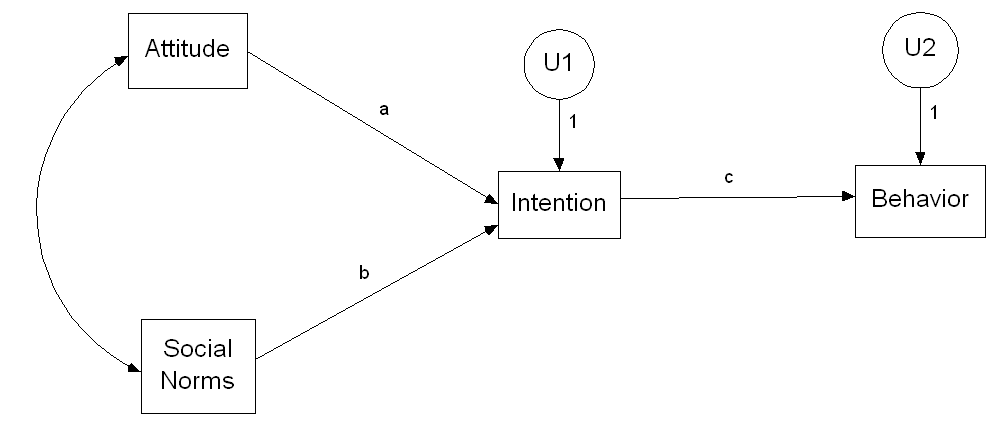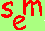David A. Kenny
August 15, 2011

Path Analysis

This page discusses how to use multiple regression to estimate the parameters of a structural model.

Key Assumption
For an endogenous variable, its disturbance must be uncorrelated with all of the specified causal variables.  So for a model, consider each endogenous variable and determine that its disturbance is uncorrelated with each of its causes.

Violation of the Assumptions to Use Multiple Regression to Estimate Structural Coefficients
There are three conditions under which the disturbance is correlated with the exogenous variable (thus, eliminating multiple regression as an appropriate tool to estimate causal paths):
a) Spuriousness:  A variable causes both the endogenous variable and one its causal variables and that variable is not included in the model.
b) Reverse Causation:  The endogenous variable causes, either directly or indirectly, one of its causes.
c) Measurement Error:  There is measurement error in a causal variable.

Estimation Using Multiple Regression

Unstandardized variables
Paths: b weights from the regression equation
disturbance variance: the variance of the endogenous variable times one minus the multiple correlation squared
Curved lines between exogenous variables: covariances
Curved lines between disturbances: partial covariances, with causal variables of both endogenous variables partialled

Standardized variables
Paths: beta weights from the regression equation
Disturbance Path: square root of one minus the multiple correlation squared
Curved lines between exogenous variables: correlations
Curved lines between disturbances: partial correlations with common causal variables of both endogenous variables partialled

Steps in Testing
STEP ONE: TEST OF DELETED PATHS
Respecify the model to make it just-identified or saturated.  That is, add the paths that the model specifies to be zero and include them in the model.  Test the paths specified to be zero making sure that the specified paths are included in the equation, but not tested.   These tests may be done with a reduced alpha (e.g., .01).
STEP TWO: TEST OF SPECIFIED PATHS
Retaining the significant paths from the previous step, test the paths that were specified to be present in the   Sometimes these tests are done hierarchically.
STEP THREE: TRIMMED MODEL
Re-estimate the model, including (a) the paths that were specified to be zero but were significant from step one and (b) dropping the paths that were specified but were not significant in step two.  In some cases, the model should not be trimmed if either the goal is to compare the paths from one model to another or if the goal is determine the absolute size of the paths.

Types of Tests
a) test of the individual paths: standard t or F test of the coefficient F
b) test of all of the paths in a given equation

(N - p - 1)(R22 - R12)
________________
k(1 - R22)

where N is the overall sample size, p is the number of deleted plus specified paths, k is the number of deleted paths, R12  is the multiple correlation squared (not adjusted) from the equation with only the specified paths, and R22  is the multiple correlation squared (not adjusted) from the equation with the specified and deleted paths.
c) The combined test of all of the paths in the model is usually a chi square goodness of fit test from a SEM program such as AMOS, EQS, MPLUS, or LISREL.

Determination of Deleted Paths
If all of the paths in the model can be estimated by multiple regression, the number of deleted paths equals the number of knowns minus the number of unknowns or the degrees of freedom of the specified model.  To make the model just-identified, examine each pair of variables and determine pairs of variables that are not linked by a path or a correlation (including a correlation between disturbances).  Add a path or a correlation between disturbance between each pair of these unlinked pairs.  The direction of the path is given by theory and the requirement that feedback not be introduced.

Types of Links Between Two Variables
Direct effect:  Either X causes Y, Y causes X, or both.

Indirect effect:  The relationship between X and Y is said to be indirect if X causes Z which in turn causes Y. (To learn more about indirect effects and mediation.)

Spuriousness:  The relationship between X and Y is said to be spurious  if Z causes X and Y.

Unexplained covariation:  Both X and Y are exogenous and so variation  between them is not explained by the model.  Other names for spuriousness are omitted variables, confounding, and third-variable causation.

Decomposition of a Correlation
Correlation between two endogenous variables:

Correlation = Direct Effect + Indirect Effects + Spuriousness

Correlation between an endogenous variable and an exogenous variable:

Correlation = Direct Effect + Indirect Effects + Unspecified Covariance

Example
Below is the Theory of Planned Behavior of Fishbein and Ajzen.The coefficients in this model can be estimated by multiple regression:  For the Intention equation, it disturbance, U1 is uncorrelated with both Attitude and Social Norms and for the Behavior equation, it disturbance, U2 is uncorrelated with both Intention.

There are two deleted paths model: One from Attitude to Behavior and the other from Social Norms to Behavior.  If these two paths are added to the model, it would be saturated.  The following three steps are suggested:

STEP ONE: TEST OF DELETED PATHS
We add the paths from Attitude and Social Norms to Behavior.   We test these paths including the specified path of Intention to Behavior.
STEP TWO: TEST OF SPECIFIED PATHS
Retaining any of the significant paths from the previous step, we test the paths a, b, and c.
STEP THREE: TRIMMED MODEL
Because this is a fairly standard model, we probably would not trim.  If we did and say found that path b, the path from Social Norms to Intention, was not needed, we re-estimate the model with that path dropped and then report the resulting coefficients.

Go to the next SEM page.Go to the SEM page.